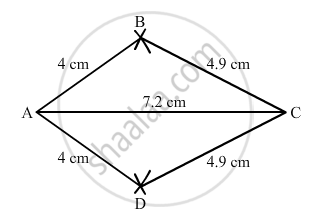Advertisement Remove all ads

# Construct a Kite Abcd in Which Ab = 4 Cm, Bc = 4.9 Cm and Ac = 7.2 Cm. - Mathematics

Answer in Brief

Construct a kite ABCD in which AB = 4 cm, BC = 4.9 cm and AC = 7.2 cm.

Advertisement Remove all ads

#### Solution

$\text { Steps of construction }:$

$\text { Step I: Draw AC = 7 . 2 cm } .$

$\text { Step II: With A as the centre and radius 4cm, draw arcs on both sides of the line segment AC }.$

$\text { Step III : With C as the centre and radius 4 . 9 cm, draw arcs on both sides of AC intersecting the previous arcs of step II at B and D }.$

$\text { Step IV: Join BA, DA, BC and CD .}$

$\text { Thus, the quadrilateral ABCD so obtained is the required kite } .$Is there an error in this question or solution?
Advertisement Remove all ads

#### APPEARS IN

RD Sharma Class 8 Maths
Chapter 18 Practical Geometry (Constructions)
Exercise 18.1 | Q 6 | Page 4
Advertisement Remove all ads

#### Video TutorialsVIEW ALL 

Advertisement Remove all ads
Share
Notifications

View all notifications

Forgot password?
Course